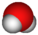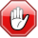TIP4P/2005 model of waterThis article is a 'stub' about water and/or ice. It has no, or next to no, content. It is here at the moment to help form part of the structure of SklogWiki. If you add material to this article, remove the {{Stub-water}} template from this page.This page contains numerical values and/or equations. If you intend to use ANY of the numbers or equations found in SklogWiki in any way, you MUST take them from the original published article or book, and cite the relevant source accordingly.

The TIP4P/2005 model is a re-parameterisation of the original TIP4P potential for simulations of water. TIP4P/2005 is a rigid planar model, having a similar geometry to the Bernal and Fowler (BF) model.

Parameters$r_{\mathrm {OH}}$ (Å)$\angle$HOH , deg$\sigma$ (Å)$\epsilon/k$ (K) q(O) (e) q(H) (e) q(M) (e)$r_{\mathrm {OM}}$ (Å) 0.9572 104.52 3.1589 93.2 0 0.5564 -2q(H) 0.1546

Surface tension

The surface tension has also been studied for the TIP4P/2005 model (Ref. 3).

Self-diffusion coefficient

The TIP4P/2005 potential has a self-diffusion coefficient, in bulk water at 298 K, of 0.21 Å2 ps−1 in a classical simulation of 216 water molecules (Ref. 4) (experimental value: 0.23 Å2 ps−1).